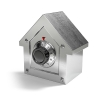#### You may also like### Always Two

Find all the triples of numbers a, b, c such that each one of them plus the product of the other two is always 2.### Symmetricality

Five equations and five unknowns. Is there an easy way to find the unknown values?### A Change in Code

Can you find the percentage increase if the results appear in code?

# Continuing to Explore Four Consecutive Numbers

##### Age 14 to 16Challenge Level

This problem follows on from, Starting to Explore Four Consecutive Numbers.

Take four consecutive numbers, $a$, $b$, $c$, $d$.

1. Why can't $bd-ac$ be even?

2. What is $bc-ad$ always equal to?

3. Why must the sum $a+b+c+d$ have an odd factor?

4. Why can't the sum $a+b+c+d$ be a multiple of $4$?

5. Which consecutive numbers are such that $a+b+c+d$ divides exactly by $3$?

If you enjoyed working on this problem, you may now want to take a look at the follow-up problem, Is There More to Discover About Four Consecutive Numbers?

With thanks to Don Steward, whose ideas formed the basis of this problem.